# 并查集——解决连接问题的神器1 定义

2 Quick Find实现

3 Quick Union

4 基于size的优化

5 基于rank的优化

6 路径压缩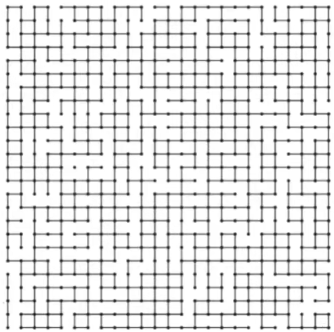``````// 将两个数据合并起来
union(p,q);``````
``````// 判断p，q是否相连
isConnected(p,q);``````

``````public interface UF {
int getSize();
boolean isConnected(int p, int q);
void unionElements(int p, int q);
}``````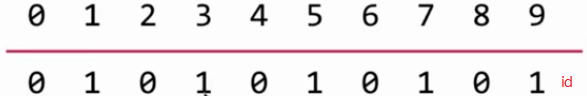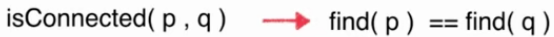``````public class UnionFind1 implements UF {

private int[] id;    // 本质就是一个数组

public UnionFind1(int size) {
id = new int[size];
// 初始化, 每一个id[i]指向自己, 没有合并的元素
for (int i = 0; i < size; i++)
id[i] = i;
}

@Override
public int getSize(){
return id.length;
}

// 查找元素p所对应的集合编号
// O(1)复杂度
private int find(int p) {
if(p < 0 || p >= id.length)
throw new IllegalArgumentException("p is out of bound.");
return id[p];
}

// 查看元素p和元素q是否所属一个集合
// O(1)复杂度
@Override
public boolean isConnected(int p, int q) {
return find(p) == find(q);
}

// 合并元素p和元素q所属的集合
// O(n) 复杂度
@Override
public void unionElements(int p, int q) {
int pID = find(p);
int qID = find(q);
if (pID == qID)
return;
// 合并过程需要遍历一遍所有元素, 将两个元素的所属集合编号合并
for (int i = 0; i < id.length; i++)
if (id[i] == pID)
id[i] = qID;
}
}``````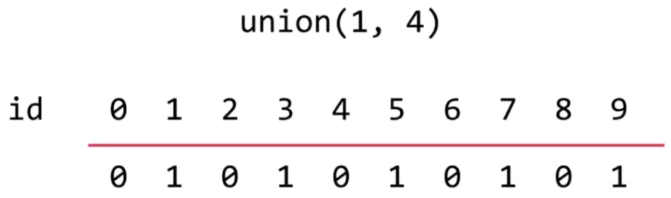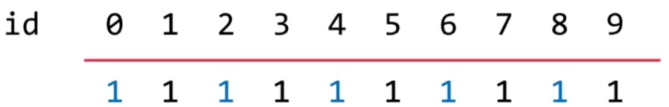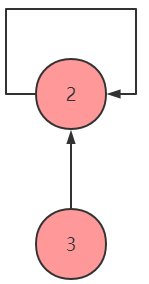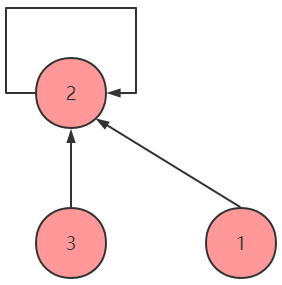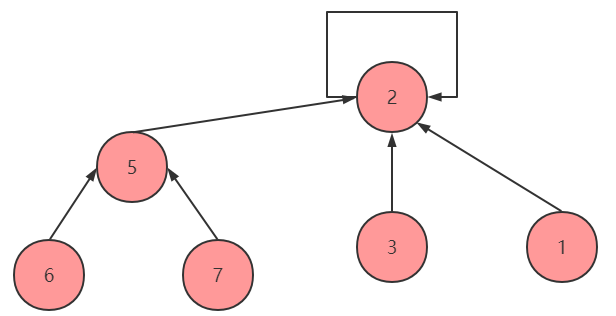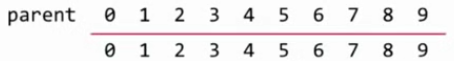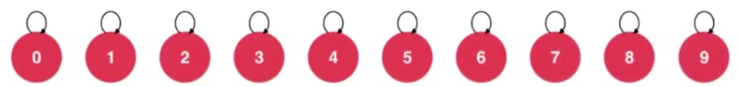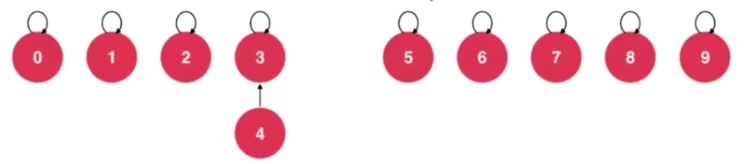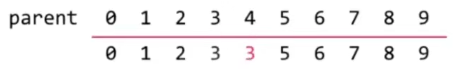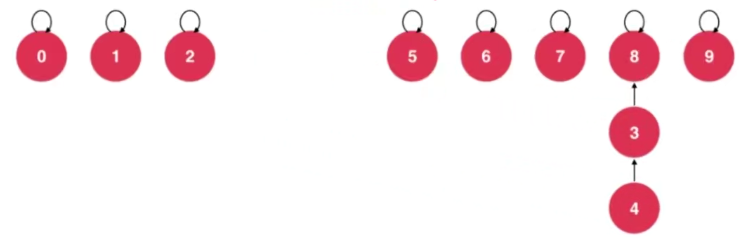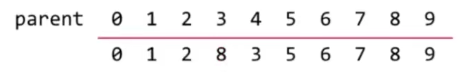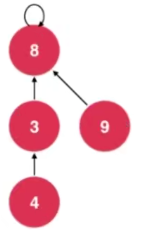union过程：O(h)，其中h为树的高度，h << n。相应的查询过程的时间复杂度也是O(h)。

``````public class UnionFind2 implements UF {

// 使用一个数组构建一棵指向父节点的树
// parent[i]表示第一个元素所指向的父节点
private int[] parent;

// 构造函数
public UnionFind2(int size){
parent = new int[size];
// 初始化, 每一个parent[i]指向自己, 表示每一个元素自己自成一个集合
for( int i = 0 ; i < size ; i ++ )
parent[i] = i;
}

@Override
public int getSize(){
return parent.length;
}

// 查找过程, 查找元素p所对应的集合编号
// O(h)复杂度, h为树的高度
private int find(int p){
if(p < 0 || p >= parent.length)
throw new IllegalArgumentException("p is out of bound.");
// 不断去查询自己的父亲节点, 直到到达根节点
// 根节点的特点: parent[p] == p
while(p != parent[p])
p = parent[p];
return p;
}

// 查看元素p和元素q是否所属一个集合
// O(h)复杂度, h为树的高度
@Override
public boolean isConnected( int p , int q ){
return find(p) == find(q);
}

// 合并元素p和元素q所属的集合
// O(h)复杂度, h为树的高度
@Override
public void unionElements(int p, int q){
int pRoot = find(p);
int qRoot = find(q);
if( pRoot == qRoot )
return;
parent[pRoot] = qRoot;
}
}``````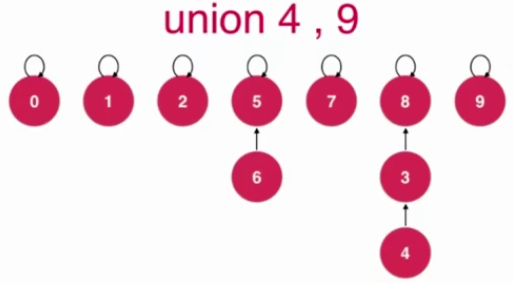``````public void unionElements(int p, int q){
int pRoot = find(p);
int qRoot = find(q);
if(pRoot == qRoot)
return;

// 根据两个元素所在树的元素个数不同判断合并方向
// 将元素个数少的集合合并到元素个数多的集合上
if(sz[pRoot] < sz[qRoot]){
parent[pRoot] = qRoot;
sz[qRoot] += sz[pRoot];
}
else{ // sz[qRoot] <= sz[pRoot]
parent[qRoot] = pRoot;
sz[pRoot] += sz[qRoot];
}
}``````

rank指树的高度。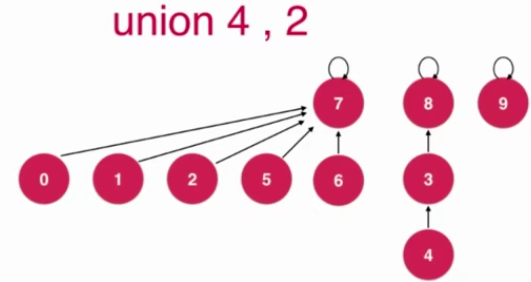``````public void unionElements(int p, int q){
int pRoot = find(p);
int qRoot = find(q);

if( pRoot == qRoot )
return;

// 根据两个元素所在树的rank不同判断合并方向
// 将rank低的集合合并到rank高的集合上
if(rank[pRoot] < rank[qRoot])
parent[pRoot] = qRoot;
else if(rank[qRoot] < rank[pRoot])
parent[qRoot] = pRoot;
else{ // rank[pRoot] == rank[qRoot]
parent[pRoot] = qRoot;
rank[qRoot] += 1;   // 此时维护rank的值
}
}``````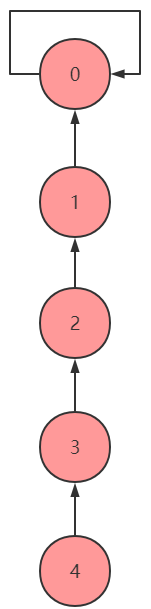``parent[p] = parent[parent[p]];``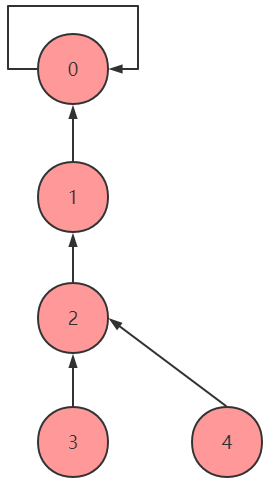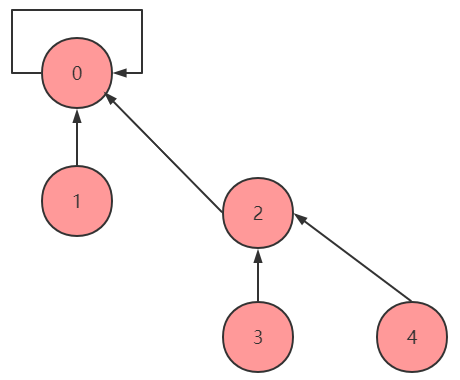``````private int find(int p){
if (p < 0 || p >= parent.length)
throw new IllegalArgumentException("p is out of bound");

while (p != parent[p]){
parent[p] = parent[parent[p]];  // 路径压缩，添加后rank不再表示树的高度的物理意义，但是依然有效
p = parent[p];
}
return p;
}``````

``````/*
quick union

*/
public class UnionFind implements UF{
private int[] parent;
private int[] rank;   // sz[i]表示以i为根的集合中元素个数

public UnionFind(int size){
parent = new int[size];
rank = new int[size];
for (int i = 0; i < size; i ++){
parent[i] = i;
rank[i] = 1;
}
}

@Override
public int getSize(){
return parent.length;
}

// 查找过程，查找p对应的集合编号
// O(h)，h为树高度
private int find(int p){
if (p < 0 || p >= parent.length)
throw new IllegalArgumentException("p is out of bound");

while (p != parent[p]){
parent[p] = parent[parent[p]];  // 路径压缩，添加后rank不再表示树的高度的物理意义，但是依然有效
p = parent[p];
}
return p;
}

@Override
public boolean isConnected(int p, int q){
return find(p) == find(q);
}

// 合并过程，合并pq所属的集合
// O(h)，h为树高度
@Override
public void unionElements(int p, int q){
int pRoot = find(p);
int qRoot = find(q);

if (pRoot == qRoot)
return;
// 让rank低的树的根节点指向rank高的树的根节点
if (rank[pRoot] < rank[qRoot]){
parent[pRoot] = qRoot;
// 高度低的树的根指向高度高的树的根，总的高度不变不用维护rank
}
else if (rank[qRoot] < rank[pRoot]){
parent[qRoot] = pRoot;
}
else {  // rank[qRoot] == rank[pRoot]
parent[qRoot] = pRoot;
rank[pRoot] += 1;
}
}
}``````

-- 展开阅读全文 --

« 上一篇 07-24